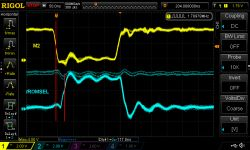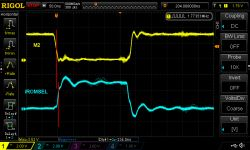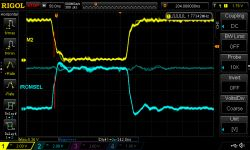## falling edge M2 and rising edge /ROMSEL issues delay issues

Discuss hardware-related topics, such as development cartridges, CopyNES, PowerPak, EPROMs, or whatever.

Moderators: B00daW, Moderators

krzysiobal
Posts: 755
Joined: Sun Jun 12, 2011 12:06 pm
Location: Poland

### falling edge M2 and rising edge /ROMSEL issues delay issues

While all of us knows that there is slight delay between rising edge of M2 and falling edge of /ROMSEL (caused by the 74138 in chip-based consoles) that can be problematic when decoding faster RAMs under \$8000,

Code: Select all

``````M2       _____-------
/ROMSEL  -------_____
``````
but it can be easily solved by delaying the rising edge of M2 by the circuit,

Code: Select all

``````M2--+--<|--+--- delayed M2
|      |
+--1k--+
|
56p
|
GND
``````
I've just recently spotted the opposite problem when after hours of digging, I've found why my cartridge did not work on SOME famiclones: the delay between falling edge of M2 and rising edge of /ROMSEL:

Code: Select all

``````M2       -----_______
/ROMSEL  _______-----
``````
1) In chip based consoles, this is equal to ~25-50ns2) In some blob ones this might be much less (like 5ns)3) Or even 0 in other weird famiclone:You might ask - why this is so important? This is because during writes, DATA bus starts to be valid around half of the CPU cycle, so the only safe way to read it is at the end of CPU cycle (just like RAMs do: they latch address at the falling edge of its /CE but data on rising edge)

Code: Select all

``````M2       -----______________--
/ROMSEL  _______------------__
DATA     -------------<VALID>-
``````

Affected use cases things:

A)
Let's consider that we are implementing mapper that has only registers at \$8000-\$ffff. Naturally, the simplest way is to interpret writes when /ROMSEL rises and don't even care for M2.

Code: Select all

``````process(...) is begin
if rising_edge(CPU_nROMSEL) and CPU_RnW='0' then  --for writes \$8000-\$ffff
...
end if;
end process;
``````
B)
Another type of mapper is when it has registers only below \$8000, then it would be like:

Code: Select all

``````process(...) is begin
if falling_edge(M2) and CPU_nROMSEL='1' and CPU_RnW='0' then  --for writes \$0000-\$7fff
...
end if;
end process;
``````
C)
Yet another type of mapper is when it has both registers under and above \$8000, but they do not affect the same bits - we can have two separate processes just like above. This is of course starts to be quite messy because this separates our code to two places.

Code: Select all

``````process(CPU_nROMSEL) is begin
if rising_edge(CPU_nROMSEL) and CPU_R_nW='0' then  --for writes \$8000-\$ffff
...
end if;
end process;

process(CPU_M2) is begin
if falling_edge(CPU_M2) and CPU_nROMSEL='1' and CPU_R_nW='0' then  --for writes \$0000-\$7fff
...
end if;
end process;
``````
D)
But what if we need to deal with mapper that affect the same bits for both under and over \$8000? For example:

Code: Select all

``````\$0000-\$ffff [.......V] - set some fancy `foo` registers to value V
``````
We can't do it like that

Code: Select all

``````process(CPU_nROMSEL) is begin
if rising_edge(CPU_nROMSEL) and CPU_R_nW='0' then  --for writes \$8000-\$ffff
foo <= CPU_D(0);
end if;
end process;

process(CPU_M2) is begin
if falling_edge(M2) and CPU_nROMSEL='1' and CPU_R_nW='0' then  --for writes \$0000-\$7fff
foo <= CPU_D(0);
end if;
end process;

beause VHDL tool would complain:
Error (10028): Can't resolve multiple constant drivers for net "foo" at cart_krzysiocart_impr.vhd(576)
``````
Merging them into single process:

Code: Select all

``````	process(CPU_nROMSEL) is begin
if rising_edge(CPU_nROMSEL) and CPU_RnW='0' then  --for writes \$8000-\$ffff
foo <= CPU_D(0);
end if;

if falling_edge(CPU_M2) and CPU_nROMSEL='1' and CPU_R_nW='0' then  --for writes \$0000-\$7fff
foo <= CPU_D(0);
end if;
end process;

wouldn't work either:
Error (10820): Netlist error at cart_krzysiocart_impr.vhd(574): can't infer register for foo because its behavior depends on the edges of multiple distinct clocks
``````

So the way I was working around that for years was to make only one process responsible for latching the value of foo and if the other wants do latch it as well, then it uses extra signals (proc1_please_latch, proc1_latch_done, proc1_latch_value) to communicate to the other one to do it behalf him:

Code: Select all

``````	process(CPU_nROMSEL) is begin
if rising_edge(CPU_nROMSEL) then
if proc1_latch_done = '1' then
end if;

if CPU_R_nW='0' then  --for writes \$8000-\$ffff
proc1_latch_value <= cpu_d(0);
end if;
end if;
end process;

process(CPU_M2) is begin
if falling_edge(CPU_M2) then
foo <= proc1_latch_value;
proc1_latch_done <= '1';
else
proc1_latch_done <= '0';
end if;

if CPU_nROMSEL='1' and CPU_R_nW='0' then  --for writes \$0000-\$7fff
foo <= '1';
end if;
end if;
end process;
``````
For larger processes it starts to be much less readable and overcomplicated. This problem is especially common when dealing with IRQs - one piece of process (dependent on the edge of PPU_A12) sets IRQ asserted signal, and other process (dependend on the edge of CPU_/ROMSEL) clears IRQ asserted signal.

So my recent idea for both simplifying the problem and making whole code to be put into one place was to aggregate both writes and watch for then when CPU_M2 falls and treat CPU_nROMSEL at that time just like it would be inverted A15:

Code: Select all

``````	process(CPU_nROMSEL) is begin
if falling_edge(CPU_M2) and CPU_R_nW='0' then
if CPU_nROMSEL='1' then  --for writes \$0000-\$7fff
foo <= CPU_D(0);
else                     --for writes \$8000-\$ffff
foo <= CPU_D(0);     -- (*)
end if;
end if;
end process;
``````
and this is where I spotted my problem on SOME famiclones - the branch marked with (*) did not executed because M2 and /ROMSEL both changed at the same time.

So there are 2 solutions:
1) externally delay the rising edge of CPU_nROMSEL by the same circuit as on beginning of the post
or
2) write it in non-edge way:

Code: Select all

``````	process(CPU_nROMSEL) is begin
if CPU_M2 = '1' and CPU_R_nW = '0' then
if CPU_nROMSEL='1' then  --for writes \$0000-\$7fff
foo <= CPU_D(0);
else                     --for writes \$8000-\$ffff
foo <= CPU_D(0);
end if;
end if;
end process;
``````
Althought, I would be sceptical about the second approach because this makes the foo-latch transparent when the condition is meet (so during the whole M2 cycle) so the foo value can be toggling many times during the single cycle, possibly causing some non-intentional behaviour if this signal clocks some more internal logic.

Do you have other ideas?